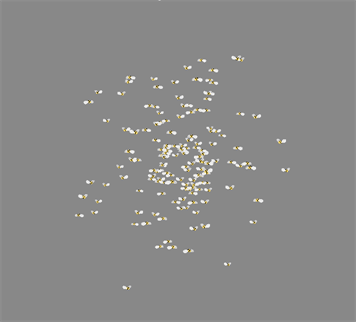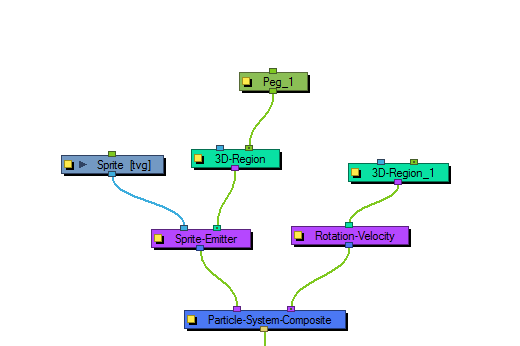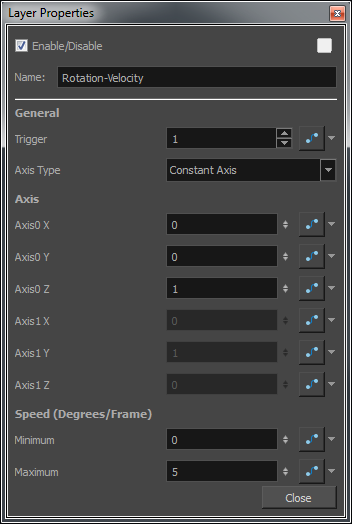# Rotation VelocityThe Rotation Velocity effect lets you define the initial rotational velocity of the particles. The values that you set up for this node refer to each individual particle's drawing axes. This means that if you choose to rotate around the X-axis, it will rotate around each particle's local X-axis as defined by its drawing plane.

The rotational velocity should be set before you emit the particles, such as to the right, so the velocity is taken into account when the particles are created.### Rotation Velocity Properties

Parameter Description
Name Allows you to change the name given to the node.
General

Trigger: An on/off switch using binary code. 1 = on, 0 = off.

Axis Type: Lets you select the type of axis to use.

 • Use Input Shape: Uses a region to define the rotational axis of the particles. The axis of each particle's rotation will be perpendicular to the plane that particle is created on.
 • Constant Axis: If you choose to rotate the particles around a constant axis, then each particle will rotate around the same axis. This axis will then be defined as Axis0.
 • Interpolated Axis: If you choose to rotate the particles around an interpolated axis, that means each particle will have its own rotational velocity which lies somewhere between Axis0 and Axis1.
Axis

Axis0 X: Defines the X component of Axis0. If you set the X to 1, and the Y and Z to 0, then the particle will rotate around its X-axis as defined by its drawing plane. If you set X to 1 and also Y to 1, then it will rotate around an axis that is 45 degrees between X and Y.

Axis0 Y: Defines the Y component of Axis0.

Axis0 Z: Defines the Z component of Axis0.

Axis1 X: Defines the X component of Axis1. This applies only when you have selected an Interpolated axis.

Axis1 Y: Defines the Y component of Axis1.

Axis1 Z: Defines the Z component of Axis1.

Speed (Degrees/Frame)

Minimum: The minimum initial rotation that a particle can have, in degrees per frame.

Maximum: The maximum initial rotation that a particle can have, in degrees per frame.